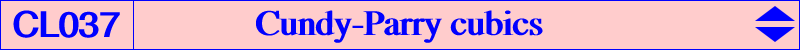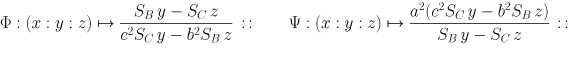H. M. Cundy and C. F. Parry have written several papers on cubics (see the bibliography) and, in particular, have studied a transformation we shall call the Cundy-Parry transformation Phi : M -> M1 = OM /\ HM*, where M* is the isogonal conjugate of M. Naturally, we can also define the related other transformation Psi : M -> M2 = HM /\ OM*. These two transformations yield a large number of interesting facts concerning cubics. They are given by :Properties of Phi and Psi   Phi and Psi are 3rd degree transformations. Phi and Psi are both involutions i.e. Phi(Phi(M)) = Psi(Psi(M)) = M. Phi(Psi(M)) = Psi(Phi(M)) = M*. Phi(M*) = Phi(M)* = Psi(M) and Psi(M*) = Psi(M)* = Phi(M). Phi(M) = M if and only if M lies on the Orthocubic K006 and Psi(M) = M if and only if M lies on the McCay cubic K003. Phi and Psi have singular points A, B, C, O, H (O is double for Phi, H is double for Psi).Phi and Psi transforms of a line L and a circum-conic L*   In the most general case, L is transformed into a nodal (with node O) circum-cubic K1 under Phi and a nodal (with node H) circum-cubic K2 under Psi. It is clear that K1 and K2 are isogonal conjugates and both contain O and H. If L* denotes the circum-conic which is the isogonal transform of the line L then K2 = Phi(L*) and K1 = Psi(L*). When L passes through a singular point, K1 and K2 decompose. For example : - if L passes through H, K1 is the union of the Euler line and a circum-conic through O, - if L passes through A, K1 is the union of the line OA and a conic through B, C, O, H, - if L passes through O, K1 is the union of the Jerabek hyperbola and the same line L through O, - if L is the Euler line, K1 is the union of the Jerabek hyperbola and the Euler line.   Special cubics K1 The nodal tangents are perpendicular if and only if L contains X(924) i.e. L is the line at infinity (in which case K1 is the Lemoine cubic K009) or is a line parallel to the lines X(50)-X(647), X(66)-X(879), X(669)-X(684), etc. K1 is a K0 (i.e. a cubic without term in xyz) if and only if L contains X(647), the perspector of the Jerabek hyperbola. K1 is an isocubic if and only if L contains X(185). In this case, it is a non-degenerate nK with pole X(577). (K1 being a nodal cubic cannot be a pK. When L contains O or H, the isocubic degenerates). K1 is circular if and only if L is the line X(5)-X(523), the perpendicular at the nine-point center to the Euler line and the trilinear polar of X(94). K1 is equilateral if and only if L is the line X(526)-X(1511), the trilinear polar of X(323). K1 is a K+ if and only if the isotomic conjugate of the tripole of L lies on a cubic through X(76) and X(877). In the first case, L is the Lemoine axis and in the second case, L is the line X(125)-X(647). In both cases, the cubic is a K0+ since these two lines contain X(647). K1 has a cusp at O if and only if the isogonal conjugate of the tripole of L lies on the parabola with focus X(112), directrix the line HK. When L is the line X(6)X(25), we obtain the cubic K737.   Special cubics K2   The nodal tangents are perpendicular if and only if L contains X(520) i.e. L is the line at infinity (in which case K2 is the Musselman cubic K028) or is a line parallel to the trilinear polar of O. K2 is a K0 (i.e. a cubic without term in xyz) if and only if L contains X(647), the perspector of the Jerabek hyperbola. K2 is an isocubic if and only if L contains X(185). In this case, it is a non-degenerate nK with pole X(393). (K2 being a nodal cubic cannot be a pK. When L contains O or H, the isocubic degenerates). K2 is circular if and only if L is the line X(5)-X(523), the perpendicular at the nine-point center to the Euler line and the trilinear polar of X(94). K2 is equilateral if and only if L is the line at infinity and this is K028. K2 is a K+ if and only if the isotomic conjugate of the tripole of L lies on a cubic through X(2) in which case L the line at infinity and K2 is K028. K2 has a cusp at H if and only if K1 has a cusp at O as above.Phi and Psi transforms of non circum-conics   In general, the Phi or Psi transform of a conic is a sextic but when the conic contains O and H, both transforms are circum-cubics with nodes at O and H respectively. See K389 for example.Phi and Psi transforms of circum-cubics   In the most general case, Phi and Psi transform a circum-cubic into a sextic. It is more interesting to consider circum-cubics passing through O and H which will transform into other circum-cubics passing through O and H.   Phi and Psi transforms of the cubics of the Euler pencil An isogonal pK with pivot P on the Euler line is a member of the Euler pencil. See Table 27. It always contains the in/excenters, O, H, P and its isogonal conjugate P* on the Jerabek hyperbola. Phi and Psi both transform this pK into another pK of the same pencil whose pivot Q is the harmonic conjugate of P with respect to O and H. Psi fixes the McCay cubic and Phi fixes the Orthocubic. Under Psi and Phi, these two cubics are globally invariant. The following table gives examples of such cubics with centers X(i) on the cubics.P pK Q Phi/Psi transform of pK X(30) K001 X(5) K005 Napoleon cubic X(2) K002 X(20) K004 Darboux cubic X(3) K003 X(3) K003 McCay cubic X(4) K006 X(4) K006 Orthocubic X(5) K005 X(30) K001 Neuberg cubic X(20) K004 X(2) K002 Thomson cubic X(384) K020 X(5999) K422 X(27) K109 X(7513) i = 1, 3, 4, 580, 581 X(10996) K152 X(7386) i = 1, 3, 4 X(5059) K156 X(5056) i = 1, 3, 4 X(376) K243 X(631) i = 1, 3, 4, 631 X(21) i = 1, 3, 4, 21, 65 X(411) i = 1, 3, 4, 411 X(24) i = 1, 3, 4, 24, 68 X(378) i = 1, 3, 4, 378 X(29) i = 1, 3, 4, 29, 73 X(412) i = 1, 3, 4, 412, 1715 X(140) i = 1, 3, 4, 140, 1173 X(550) i = 1, 3, 4, 550 X(381) i = 1, 3, 4, 381 X(382) i = 1, 3, 4, 382 X(548) i = 1, 3, 4, 548 X(549) i = 1, 3, 4, 549Phi-transform of a pK(W,O) passing through H A pK(W,O) passing through H must have its pole W on the line HK. All these cubics form a pencil since they all contain A, B, C, O, H, X(1075) and the vertices of the cevian triangle of O. The Psi-transform of such pK(W,O) is another pK with : pole a point on the circum-conic through K and X(287) whose perspector is the point a^4(b^2 - c^2) SA^2 : : , pivot a point on the circum-conic through O and X(95) whose perspector is the point a^4(b^2 - c^2) SA^3 : : .   Phi-transform of a pK(W,H) passing through O A pK(W,H) passing through O must have its pole W on the line OK. All these cubics form a pencil since they all contain A, B, C, O, H, X(155) and the vertices of the orthic triangle. The Psi-transform of such pK(W,H) is another pK with same pivot and pole another point on the line OK. The cubic is invariant if and only if W is the orthocenter or the point X(1609).   Phi / Psi-transform of a nK(X6, R, X3) passing through O and H Any nK(X6, R, X3) is globally invariant under both transformations. See CL062.Phi and Psi transforms of other curves Q150 is a quintic invariant under Phi. Q151 is a quintic invariant under Psi.